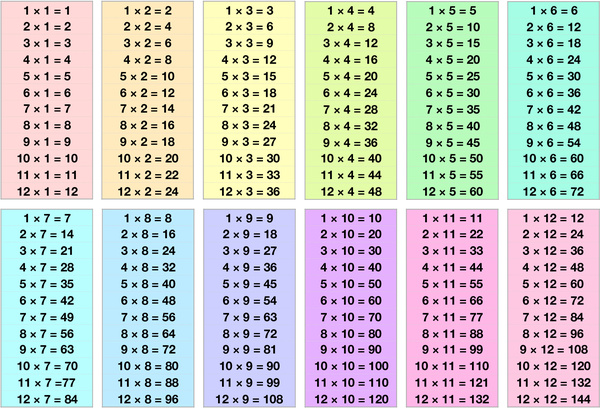# Multiplication Table 12 by 12Multiplication is a way of adding the same number over and over again. For example, 3 x 4 means adding 3 four times: 3 + 3 + 3 + 3 = 12. Learning the multiplication tables up to 12 by 12 means memorizing the answers to multiplication problems from 1 x 1 up to 12 x 12. Learning the times tables up to 12 by 12 is important for children for several reasons:

Prepares for more advanced math: As children progress through their education, they will encounter more advanced mathematical concepts that require a solid understanding of multiplication. Knowing the times tables up to 12 by 12 prepares them for this.

Increases mental agility: By knowing the times tables up to 12 by 12, children can do mental math more quickly and efficiently. This can be especially helpful in situations where they don't have access to a calculator or other tools.

Enhances problem-solving skills: Multiplication is a critical component of problem-solving, and knowing the times tables up to 12 by 12 allows children to solve more complex mathematical problems.

Improves confidence: When children have a solid understanding of multiplication, they are more likely to feel confident in their mathematical abilities. This can positively impact their academic performance and overall self-esteem.

Facilitates real-world applications: Knowing the times tables up to 12 by 12 is helpful in many real-world situations, such as calculating tips, making purchases, and measuring ingredients in recipes.

In summary, learning the times tables up to 12 by 12 is an important skill for children as it prepares them for more advanced mathematical concepts, increases mental agility, enhances problem-solving skills, improves confidence, and facilitates real-world applications. As a small kid, you can start by memorizing the multiplication tables up to 5 by 5. Once you're comfortable with those, you can move on to the multiplication tables up to 10 by 10. Try practicing with flashcards, games, or worksheets to help you memorize the multiplication tables. With practice and repetition, you'll be able to quickly recall the answers to multiplication problems, which will make learning math much easier!